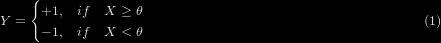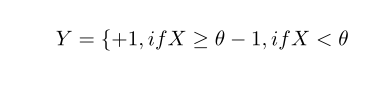# Latex换行问题\begin{equation}
Y=\left\{
\begin{aligned}
+1 & , & if & & X \geq \theta \\
-1 & , & if & & X < \theta
\end{aligned}
\right.
\end{equation}
\begin{equation}
Y=\begin{cases}